# Chemical Engineering - Chemical Reaction Engineering

Exercise : Chemical Reaction Engineering - Section 4
6.
The rate controlling step for the heterogeneous irreversible catalytic reaction A(g) + B(g)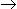C(g) is the surface reaction of absorbed A with absorbed B to give adsorbed C. The rate expression for this reaction can then be written as (where, KA, KB and KC are the equilibrium constants and is the rate constant of the rate controlling step.)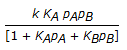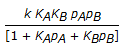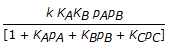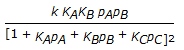Explanation:
No answer description is available. Let's discuss.

7.
The optimum performance for reactors operating in parallel is obtained when the feed stream is distributed in such a way, that the
space time for each parallel line is same.
space time for parallel lines is different.
larger reactors have more space time compared to smaller ones.
none of these.
Explanation:
No answer description is available. Let's discuss.

8.
A catalyst is said to be a negative catalyst, ifit
retards the rate of reaction.
reduces the value of equilibrium constant.
does not initiate the reaction.
all (a), (b) and (c).
Explanation:
No answer description is available. Let's discuss.

9.
For the non catalytic reaction of particles with surrounding fluid, the same needed to achive the same fractional conversion for particles of different unchanging sizes is proportional to the particle diameter, when the __________ is the controlling resistance.
film diffusion
diffusion through ash layer
chemical reaction
either (a), (b) or (c)
Explanation:
No answer description is available. Let's discuss.

10.
Pure ethanol vapor is fed to a reactor packed with alumina catalyst, at the rate of 100 kmole / hr. The reactor products comprise: ethylene :95 kmole / hr, water vapour: 97.5 k mole / hr and diethyl ether :2.5 kmole/hr. The reactions occuring can be represented by:
C2H5OHC2H4 + H2O
2C2H5OHC2H5 - O - C2H5 + H2O
The percent conversion of ethanol in the reactor is
100
97.5
95
2.5